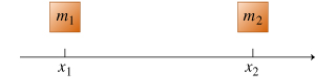# Problem: (a) Find the  x-coordinate  xcm  of the system's center of mass. Give your answer in terms of  m1,  m2,  x1, and x2.(b) If  m2  &gt;&gt;  m1 , then the center of mass is located:A. Left of  m1  at a distance much greater than  x2 - x1.B. Left of  m1  at a distance much less than  x2 - x1.C. Right of  m1 at a distance much less than  x2 - x1.D. Right of  m2 at a distance much greater than  x2 - x1.E. Right of  m2 at a distance much less than  x2 - x1.F. Left of  m2 at a distance much less than  x2 - x1.

###### FREE Expert Solution
90% (411 ratings)
###### FREE Expert Solution

(a) At the center of mass of the system:

$\overline{){\mathbf{x}}{\mathbf{=}}\frac{{\mathbf{m}}_{\mathbf{1}}{\mathbf{x}}_{\mathbf{1}}\mathbf{+}{\mathbf{m}}_{\mathbf{2}}{\mathbf{x}}_{\mathbf{2}}}{{\mathbf{m}}_{\mathbf{1}}\mathbf{+}{\mathbf{m}}_{\mathbf{2}}}}$

90% (411 ratings)###### Problem Details

(a) Find the  x-coordinate  xcm  of the system's center of mass. Give your answer in terms of  m1,  m2,  x1, and x2.

(b) If  m2  >>  m1 , then the center of mass is located:

A. Left of  m1  at a distance much greater than  x2 - x1.
B. Left of  m1  at a distance much less than  x2 - x1.
C. Right of  m1 at a distance much less than  x2 - x1.
D. Right of  m2 at a distance much greater than  x2 - x1.
E. Right of  m2 at a distance much less than  x2 - x1.
F. Left of  m2 at a distance much less than  x2 - x1